## Monday, November 25, 2013

### ATAN2 using tan^-1 and angle/ARG (Various Calculators)

ATAN2

The function atan2(y,x) is defined as:

atan2(y,x) = tan^-1 (y/x) with respect to the quadrant the point (x, y) is in. In case you didn't know, with respect to point (x, y):

(x, y) is in Quadrant I if x > 0 and y > 0
(x, y) is in Quadrant II if x < 0 and y > 0
(x, y) is in Quadrant III if x < 0 and y < 0
(x, y) is in Quadrant IV if x > 0 and y < 0

Visually:This is different from the two common calculator functions used to find the arctangent: Arctangent and Argument.

The Arctangent Function

TI and Casio Calculators*: tan^-1(y/x)
Hewlett Packard Calculators*: atan (y/x)

* The majority of them

Range (Output): -90° to 90°, -π/2 to π/2 radians

How to use Arctangent to get atan(y,x)

If the point is in quadrant I:
Use atan(y/x)

If the point is in quadrant II or III:
Use atan(y/x) + 180° in degrees mode
Use atan(y/x) + π in radians mode

If the point is in quadrant IV:
Use atan(y/x) + 360° in degrees mode
Use atan(y/x) + 2*π in radians mode

Special cases have to be used x or y is equal to 0:

If x=0 and y<0, the angle is 270° (3*π/2 radians)
If x=0 and y>0, the angle is 90° (π/2 radians)
If y=0 and x<0, the angle is 180° (π radians)
If y=0 and x>0, the angle is 360° or 0° (2*π or 0 radians)

The Argument Function

The complex number x + yi is used.

TI Calculators: angle(x + y*i)
Casio and Hewlett Packard Calculators: ARG(x + y*i)

Range: -180° to 180°, -π to π radians

How to use the Argument function to get atan2(y,x)

This is a great way to get atan2, which cleverly makes the use of complex numbers. In addition, there are a lot fewer things to remember:

If y≥0 (Quadrants I and II):
Use ARG(x+yi)*

(*The angle function if you are using a TI calculator)

If y<0 (Quadrants III and IV):
Use ARG(x+yi) + 360° for degrees mode
Use ARG(x+yi) + 2*π for radians mode

I hope this tip is helpful. Happy Thanksgiving and I am very thankful for all who have read, followed, and supported my blog over the last two years.

Eddie

This blog is property of Edward Shore. 2013

## Sunday, November 17, 2013

### HP Prime Programming Tutorial #6: Subroutines

Subroutines

This session will show how routines work in HPPL. Generally, subroutines have be declared before the main program. Declaration is important. The details of the subroutines are after the main program.

Definitely take a look at the example programs to get a better understanding.

SUB Routines for HP Prime

General Syntax:

sub(); //declare subroutines

EXPORT main()
BEGIN
commands go here, including sub()
END;

sub()
BEGIN
commands go here
END;

SUBEXAM

This is just a demonstration of how sub routines work. This program calculates one of two values:

If A is positive, then the program evaluates A. If not, the program values B instead. Where:

A = 2(x-y)/Φ + xy
B = Φ^2

and Φ = 2e^(x+y) - e^(x-y) - e^(y-x)

We will use Φ as the subroutine.

SUB1();

EXPORT SUBEXAM(X,Y)
BEGIN
LOCAL A, B;
A:=(2*(Y-X))/SUB1(X,Y)+X*Y;
B:=(SUB1(X,Y))^2;
IF A>B THEN
RETURN A;
ELSE
RETURN B;
END;
END;

SUB1(X,Y)
BEGIN
RETURN 2*e^(X+Y)-e^(X-Y)-e^(Y-X);
END;

Examples:

SUBEXAM(-4, 1) returns 21998.918189
SUBEXAM(2,3) returns 86283.2797974
SUBEXAM(-5,-6) returns 30.648061288
SUBEXAM(2,-3) returns 21810.6046664

Days Between Dates

DDAYS Using Subroutines for HP Prime: Best for 1901 to 2099

* Remember century years not divisible by 400 are NOT leap years. This program does not take this into account. If any such years are passed, subtract one day for such year manually.

Source: HP 12C Manual - Hewlett Packard

// Declare Subroutines
SUB1();
SUB2();
SUB3();

// Main program
EXPORT DDAYS(m1,d1,y1,m2,d2,y2)
BEGIN
// ΔDYS HP 12C
LOCAL x1, x2, z1, z2;
x1:=SUB1(m1); x2:=SUB1(m2);
z1:=SUB2(m1,y1); z2:=SUB2(m2,y2);
RETURN SUB3(y2,m2,d2,z2,x2)-
SUB3(y1,m1,d1,z1,x1);
END;

SUB1(X)
BEGIN
IF X≤2 THEN
RETURN 0;
ELSE
RETURN IP(.4*X+2.3);
END;
END;

SUB2(X,Y)
BEGIN
IF X≤2 THEN
RETURN Y-1;
ELSE
RETURN Y;
END;
END;

SUB3(Y,M,D,Z,X)
BEGIN
RETURN 365*Y+31*(M-1)+D+IP(Z/4)-X;
END;

(Thanks to Owitte for pointing out my typo)

Examples:
Days Between Dates:

7/3/1985 to 2/28/1995 is 3,527 days

3/14/1977 to 11/17/2013 is 13,397 days

12/10/2010 to 6/30/2014 is 1,298 days

1/5/2015 to 3/19/2227 returns 77,506 BUT this program treats 2100 and 2200 as leap years, which in reality they are not. Subtract 2 to get the correct answer of 77,504 days.

Eddie

This blog is property of Edward Shore. 2013

### HP Prime Programming Tutorial #5: STARTAPP, STARTVIEW, RGB

Today's session is about starting other apps in a program and using colors.

Defining equations in the Program Editor and Home

The equation must be a string and be stored to the appropriate designated variable.

F# is for functions of X. (Function app).
R# is for polar functions of θ. (Polar app).
U# is for sequences of N, N-1, N-2. (Sequence app).
X# and Y# for parametric equations of T. (Parametric App)
V# for open statements and equations in the Advanced Graphing App, which The independent variables are X and Y.

# is a digit 0-9.

Defining equations this way leaves them uncheck. If you want them plotted or accessed in Num View, you will need to check them.

Example:
F1:="2*X^3" stores the function f(x) = 2*x^3 in Function 1.

R5:="A*SIN(θ)" stores the polar function r(θ) = A*sin(θ) in Polar Function 5, with A being what value stored in it.

STARTAPP

STARTAPP(application name in quotes);

Starts the named App. The calculator points the screen to the default view (Plot, Symb, Num).

Access: Cmds, 4. App Functions, 2. STARTAPP

CHECK and UNCHECK

Checks and unchecks specific equation or function (0-9) in the current app. For example, if you are in the Function app, CHECK(1) activates F. As you should expect, UNCHECK(1) turns F1 off.

What does CHECK and UNCHECK affect?
1. Whether a function is plotted in Plot view.
2. Whether a function is analyzed in Num view.

Access for CHECK: Cmds, 4. App Functions, 1. CHECK
Access for UNCHECK: Cmds, 4. App Functions, 4. UNCHECK

STARTVIEW

Instructs the HP Prime to go to a certain view. It has two arguments, the view number and a redraw number.

Common view numbers include (not all inclusive):
-2 = Modes screen
-1 = Home
0 = Symbolic (Symb)
1 = Plot
2 = Numeric (Num)
3 = Symbolic Setup
4 = Plot Setup
5 = Numeric Setup
6 = App Information
7 = The Views Key
8 = first special view
9 = second special view
Etc..

The redraw number is either 0 or non-zero. 0 does not redraw the screen, anything else does. I recommend the latter.

Syntax: STARTVIEW(view number, redraw number)

Access: Cmds, 4. App Functions, 3. STARTVIEW

RGB

Returns an integer code pertaining to a color's RGB code. This is super useful for drawing and text writing.

Syntax: RGB(red, green, blue, alpha)

Red: Intensity of Red, 0-255
Green: Intensity of Green, 0-255
Blue: Intensity of Blue, 0-255
Alpha: (optional) Opacity (up to 128).

RGB codes:
Blue: RGB(0,0,255)
Violet: RGB(143,255,0)
Dark Green: RGB(0,128,0)
Orange: RGB(255,127,0)
Yellow: RGB(0,255,255)
Red: RGB(255,0,0)
White: RGB(255,255,255)
Black: RGB(0,0,0)
Gray: RGB(129,128,128)
Brown: RGB(150,75,0)
Light Blue: RGB(173,216,330)

For other colors, RGB can be found on various sites on the Internet, including Wikipedia.

Access: Cmds, 2. Drawing, 5. RGB

Tip: Change a color of a graph

Use the syntax

F#(COLOR):=RGB(red,blue,green,[alpha]);

F stands for the designated function type (F for function, R for polar, etc)
# is the digit 0-9.

Example:
F8(COLOR):=RGB(0,0,255)
makes the function F8 plot in blue.

This is a lot, but this is doable. Let's see all these commands and tips in action and create some magic.

Conic Drawing for HP Prime

Draws the conic section for the general equation

Ax^2 + By^2 + Cxy + Dx + Ey + F = 0

You can choose the color how the conic section is plotted, from red, blue, orange, and green. (Game show enthusiasts take note of the order of the colors I listed... ;) ).

EXPORT CONIC()
BEGIN
LOCAL cr, cg, cb, I;
INPUT({A,B,C,D,E,F},
"Ax^2+By^2+Cxy+Dx+Ey+F", { }, { },
{0,0,0,0,0,0});

// Colors
CHOOSE(I, "Choose a Color",
"Red","Blue","Orange","Green");
cr:={255,0,255,0};
cg:={0,0,127,255};
cb:={0,255,0,0};

V1:="A*X^2+B*Y^2+C*X*Y+D*X+E*Y+F=0";
V1(COLOR):=RGB(cr(I),cg(I),cb(I));
CHECK(1);
// Plot View
STARTVIEW(1,1);
END;

Below are some examples. Remember the form:

Ax^2 + By^2 + Cxy + Dx + Ey + F = 0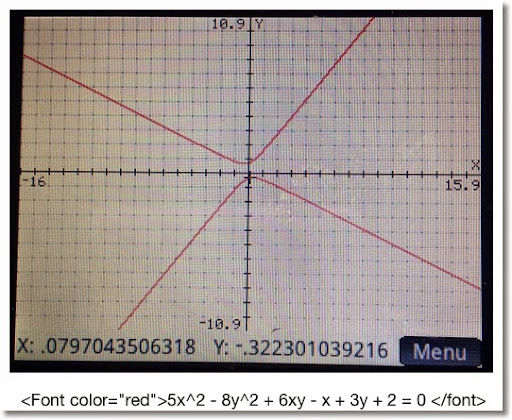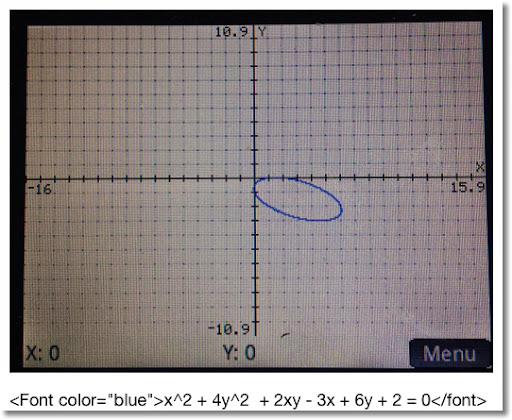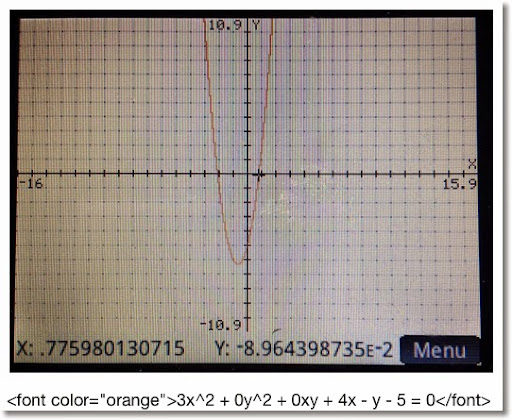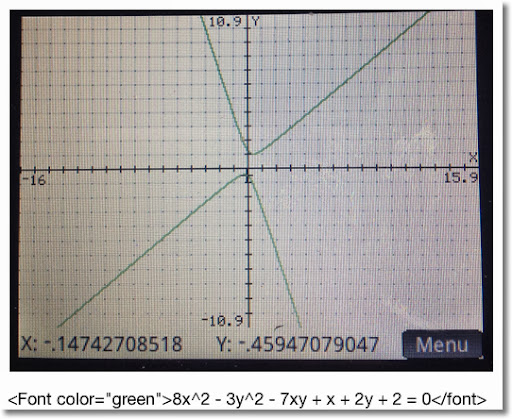Projectile Motion for HP Prime

This program calculates range and height of a projectile, and plots its path. The program sets the mode into Degrees (HAngle=1) and the calculator to the Parametric app.

Equations:
x = V * cos θ * t
y = V * sin θ * t - .5 * g * t^2

Where
V = initial velocity
θ = initial degree of flight
g = Earth gravitation constant (9.80665 m/s^2, ≈32.17404 ft/s^2)

Air resistance is not factored, so we are dealing with ideal conditions. How much the projectile represents reality varies, where factors include the object being projected, the temperate and pressure of the air, and the weather.

EXPORT PROJ13()
BEGIN
LOCAL M, str;
// V, G, θ are global
// Degrees
HAngle:=1;

CHOOSE(M, "Units", "SI", "US");
IF M==1 THEN
str:="m";
G:=9.80665;
ELSE
str:="ft";
G:=32.17404;
END;

INPUT({V, θ}, "Data",
{"V:","θ:"},
{"Initial Velocity in "+str+"/s",
"Initial Angle in Degrees"});

X1:="V*COS(θ)*T";
Y1:="V*SIN(θ)*T-.5*G*T^2";

STARTAPP("Parametric");
CHECK(1);
Xmin:=0
// Range
Xmax:=V^2/G*SIN(2*θ);
Ymin:=0
// Height
Ymax:=(V^2*SIN(θ)^2)/(2*G);
MSGBOX("Range: "+Xmax+" "+str+", "
+", Height: "+Ymax+" "+str);
// Plot View
STARTVIEW(1,1);
END;

Below are screen shots from an example with V = 35.25 m/s and θ = 48.7°.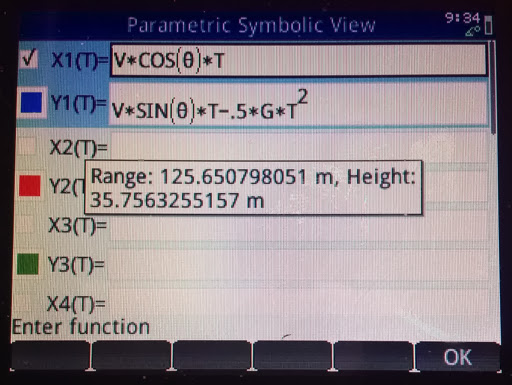This concludes this session of the tutorials. Shortly I will have Part 6 up, which has to do routines.

I am catch-up mode, still. But then again I always feel like there is too much to do and too little time. LOL

See you soon!

Eddie

This blog is property of Edward Shore. 2013

## Wednesday, November 13, 2013

### HP Prime Programming Tutorial #4: CHOOSE and CASE, Tip about INPUT

Welcome to Part 4 of our programming series for the Prime. Today's session will cover CHOOSE and CASE.

First a tip from Han of the MoHPC Forum, which is found at http://www.hpmuseum.org/cgi-sys/cgiwrap/hpmuseum/forum.cgi#255084. Thank you Han for allowing me to share this.

Use the IF THEN ELSE structure with INPUT to execute a set of default instructions if the user presses cancel. INPUT returns a value of 0 if ESC or cancel is pressed, and 1 if a value is entered.

IF INPUT(...) THEN
commands if values are entered
ELSE
commands if Cancel is pressed
END;

Default values can be assigned to values as an optional fifth argument for INPUT.

INPUT(var, "Title", "Prompt", "Help", default value)

The type of variable maybe set to other than real numbers. Just remember to store such type before the INPUT command. For example, if you want var to be a string, store an empty string:

var:=" ";

Again, major thanks to Han.
CHOOSE and CASE

CHOOSE: Creates a pop up choose box, similar to what you see when you click on a soft menu. There are two syntaxes for CHOOSE:

Simple Syntax (up to 14 options):
CHOOSE(var, "title string", "item 1", "item 2", ... , "item n");

List syntax (infinite amount of items):
CHOOSE(var, "title string", {"item 1", "item 2"});

Choosing item 1 assigns the value of 1 to var, choosing item 2 assigns the value of 2 to var.

Access: Cmds, 6. I/O, 1. CHOOSE

CASE: Allows for different test cases for one variable. Also includes a default scenario (optional).

CASE
IF test 1 THEN do if true END;
IF test 2 THEN do if true END;
...
DEFAULT commands END;

Access: Cmds, 2. Branch, 3. CASE

Let's look at two programs to demonstrate both CHOOSE and CASE.
TERMVEL - Terminal Velocity of an Object

EXPORT TERMVEL()
BEGIN

LOCAL L0:={9.80665,32.174},
L1:={1.225,.0765},
L2:={.47,1.05,1.15,.04},C,K,M,A,T;

CHOOSE(C,"Units","SI","English");

CHOOSE(K,"Type of Object","Sphere","Cube",
"Cylinder","Tear-Shaped");

INPUT({M,A},"Object",
{"M=","A="},{"Mass","Surface Area"});

T:=√((2*M*L0(C))/(L1(C)*A*L2(K)));

MSGBOX("Terminal Velocity="+T);
RETURN T;
END;

Examples:

Sphere, SI Units, M = .05 kg, A = .0028 m^2
Terminal Velocity: T = 24.6640475387 m/s

Cube, US Units, M = 1.2 lb, A = .3403 ft^2
Terminal Velocity: T = 53.149821209 ft/s

AREAC - Area of Circles, Rings, and Sectors

EXPORT AREAC()
BEGIN
LOCAL C,R,S,θ,A;
CHOOSE(C,"Areas","1. Circle","2. Ring","3. Sector");

CASE
IF C==1 THEN A:=π*R^2; END;

IF C==2 THEN
A:=π*(R^2-S^2);
END;

IF C==3
INPUT(θ, "Angle", "θ=");
\\ Assume you are in the correct angle mode
IF HAngle==1 THEN
\\ Test Angle Mode
θ:=θ*π/180;
END;
A:=θ*R^2/2;
END;

END;

MSGBOX("Area is "+A);
RETURN A;
END;

Examples

R = 2.5, r = 1.5, θ = π/4 radians or 45°

Circle: 19.6349540849
Ring: 12.5663706144
Sector: 2.45436926062

That is how, in general CHOOSE and CASE work. I thank you as always. It is so good to finally be rid of a cold and firing on all cylinders again.

Eddie

This blog is property of Edward Shore. 2013

## Tuesday, November 5, 2013

### HP Prime Programming Tutorial #3: WHILE, INPUT, KILL, REPEAT, GETKEY

This tutorial is going to cover a lot, each with some new programming commands in this series. I hope you are ready for the intensity. :)

WHILE, INPUT, KILL

HP Prime Program: TARGET. TARGET is a game where you provide a guess to get a desired number. If you miss, the calculator will tell you if number is higher and lower. At the end of the game, the calculator gives you how may picks you needed to get the target number.

WHILE: Repeat a number of commands while a specific condition is test.

WHILE condition is true DO
commands
END;

Access: Tmplt, 3. Loop, 5. WHILE

Caution: Watch your ENDs! Make sure an END is with each loop and the program itself. Press the soft key Check to check your work.

INPUT: Creates an input screen for variables. On the HP Prime, the input can asked for more than one input. TARGET demonstrates INPUT with one prompt.

One Variable:
INPUT(variable, "title", "label", "help text")
Multi-Variable:
INPUT(list of variables, "title", list of "labels", list of "help text")

Note: Pressing Cancel will store a 0 in variable. You may include code of what to do if the user presses Cancel, but it is not required.

Access: Cmds, 6. I/O, 5. INPUT

KILL: Terminates program execution. Nothing dies, I promise.

Access: Tmplt. 1. Block, 3. KILL

Program:
EXPORT TARGET()
BEGIN
LOCAL C:=0, N:=RANDINT(1,20), G:=-1;
WHILE G≠N DO
C:=C+1;
INPUT(G,"Guess?","GUESS:","1 - 20");

IF G==0 THEN
KILL;
END;

IF G < N THEN
MSGBOX("Higher");
END;

IF G > N THEN
MSGBOX("Lower");
END;

END;

MSGBOX("Correct! Score: "+C);

END;

Try it and of course, you can adjust the higher limit. Here is some thing for you to try with TARGET:

1. Add a limited amount of guesses.
2. Can you display the list of guesses?

REPEAT

ULAM Algorithm: take an integer n. If n is even, divide it by 2. If n is odd, multiply it by 3 and add 1. ULAM counts how many steps it takes to get n to 1.

REPEAT:

Access: Tmplt, 3. Loop, 6. REPEAT

Featured:
CONCAT(list1, list2): Melds list1 and list2 into one.

Access: Toolbox, Math, 6. List, 4. Concatenate

EXPORT ULAM(N)
BEGIN
LOCAL C:=1, L0:={N};

REPEAT

IF FP(N/2)==0 THEN
N:=N/2;
ELSE
N:=3*N+1;
END;

C:=C+1;
L0:=CONCAT(L0,{N});
UNTIL N==1;

MSGBOX("NO. OF STEPS="+C);
RETURN L0;
END;

Examples:

ULAM(5) returns:
Message Box: "NO. OF STEPS=6"
List: {5, 16, 8, 4, 2, 1}

ULAM(22) returns:
Message Box: "NO. OF STEPS=16"
List: {22, 11, 34, 17, 52, 26, 13, 40, 20, 10, 5, 16, 8, 4, 2, 1}

GETKEY

The next section will introduce a super-important command, GETKEY. We will be working with GETKEY over the entire series.

The Program KEYNO: The person presses key presses. Which each key press, the code returns to the terminal screen. The program terminates when the Enter key is pressed.

GETKEY: Returns the key code of last key pressed. The Prime's key map is below. (Picture is from the HP Prime User's Guide)

Access: Cmds, 6. I/O, 4. GETKEY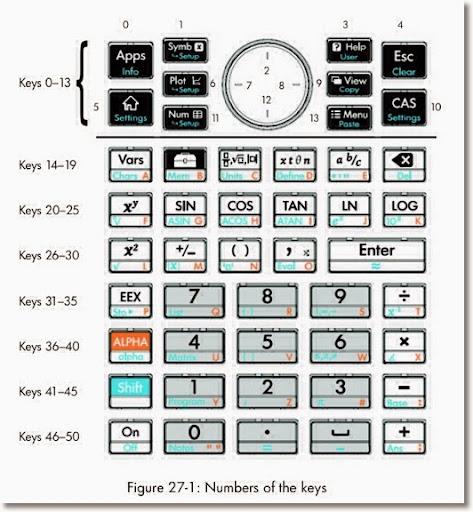EXPORT KEYNO()
BEGIN
LOCAL K;
PRINT();
PRINT("Press any key to get its code.");
PRINT("Press Enter to exit.");

REPEAT
K:=GETKEY;
IF K ≥ 0 THEN
PRINT(K);
END;
UNTIL K==30;

END;

Example Key Codes:
33: 8 key
2: up
7: left
8: right
12: down
50: plus
45: minus

This concludes Part 3. Again, it can't be said enough, thanks for all the comments and compliments. And until next time,

Eddie

This blog is property of Edward Shore. 2013

### HP Prime Tip: RPN and Created Programs

Major thanks to Miguel Toro from the MoHPC Forum for this tip:

There are two ways to call created programs in RPN mode of the HP Prime:

Vertically:
argument 1 [Enter]
argument 2 [Enter]
...
argument n [Enter]
program [Enter]

Horizontally:
argument 1 [space] argument 2 [space] ... argument n [space] program
[Enter]

Keep in mind this works with created user programs. To ensure that built-in commands work correctly, still include the number of arguments as needed.